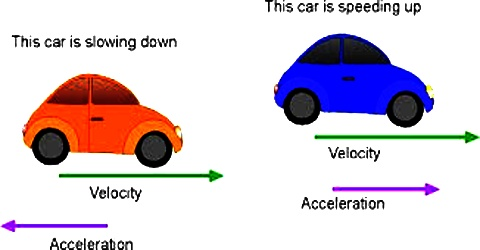# Difference between Velocity and Acceleration

Difference between Velocity and Acceleration

Velocity is the rate of displacement of an object. Acceleration is the rate of change of velocity of an object. This is the main difference. Other differences are:

Velocity

1. The rate of change of position in a definite direction is called velocity and is denoted by v.
2. If the time interval approaches zero, the rate of change of displacement with time is called velocity
3. In S.I. and M.K.S. system unit of velocity is metre/sec (ms-1)
4. Dimension of velocity = [LT-1]
5. If force is not applied on a moving body, it travels at uniform velocity along a straight line.

Acceleration

1. The rate of increase of velocity is called acceleration and is demoted by a.
2. If the time interval approaches zero, the rate of change of velocity with time is called acceleration.
3. In S.I. and M.K.S. system unit of acceleration is metre/sec2 = (ms-2).
4. Dimension of acceleration = (LT-2).
5. If force does not act on a moving body, it will have no acceleration.